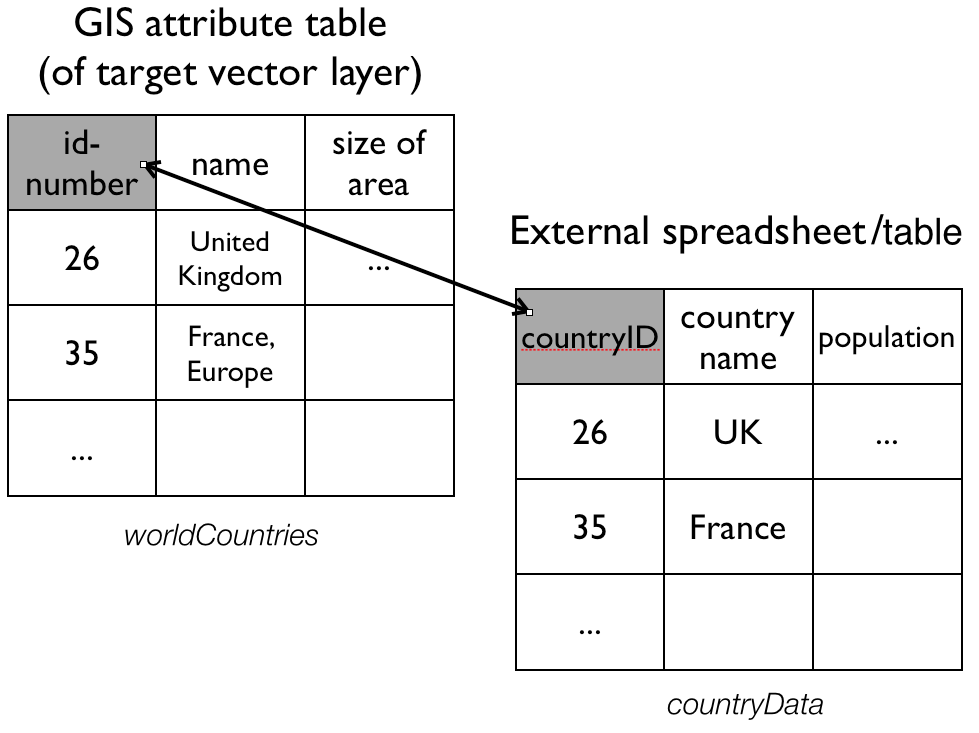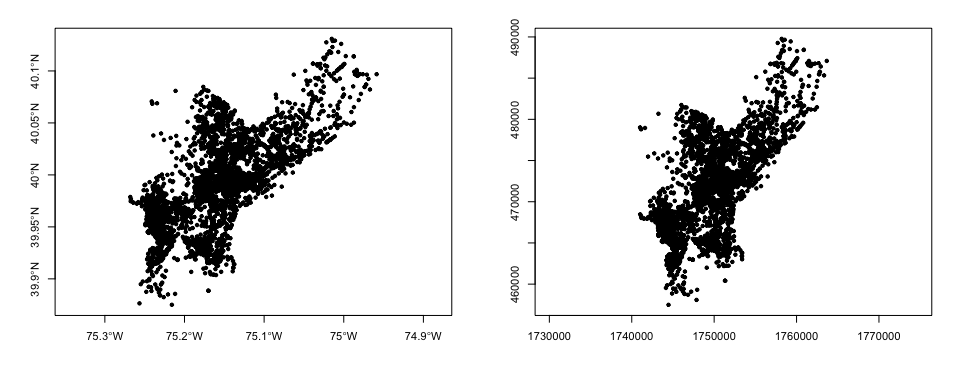In this section we will look at some libraries and commands that allow us to process vector data in R and perform a few exemplary operations.

Libraries needed:

• `sp`
• `rgdal`
• `rgeos`
• `sf`

Data needed: `RSpatialDataOps.zip`

# 1. Attribute Join

An attribute join brings tabular data into a geographic context. It refers to the process of joining data in tabular format to data in a format that holds the geometries (polygon, line, or point).

If you have done attribute joins of shapefiles in GIS software like ArcGIS or QGis you know that you need a unique identifier in both the attribute table of the shapefile and the table to be joined.

In order to combine a `Spatial*Dataframe` with another table (which would be a dataframe in R) we do exactly the same. We have a `Spatial*Dataframe`1 that contains the geometries and an identifying index variable for each. We combine it with a dataframe, that includes the same index variable with additional variables.Attribute joins in R can very simply be done with the `merge` command. Since an `sf` object is just an extension of the data frame, it works exactly as we would expect.

The `sp` package has a `merge` command which extends the one from the base package and works with Spatial* objects.

Assume we have:

• a shape file named worldCountries and
• a dataframe called countryData with the attribute data to join

where:

• “id-number” is the colum that contains the unique identifier in worldCountries, and
• “countryID” is the column that contains the unique identifier in countryData.

We would then say:

``````# for sf
worldCountries <- merge(worldCountries, countryData, by.x = "id-number", by.y = "countryID"

# for sp
require(sp) # just to make sure it is loaded
worldCountries <- merge(worldCountries, countryData, by.x = "id-number", by.y = "countryID")``````

If the names of the ID columns match, we can omit them.

Other R packages also may have convenience functions to do attribute joins. For example, the `geo_join()` command from the `tigris` package provides a convenient way to merge a data frame to a spatial data frame.

## Exercise 1

1. Download and unzip `RSpatialDataOps.zip`

2. Load the CSV table `PhillyEducAttainment.csv` (in the `nhgisPhilly_csv` folder) into a dataframe in R and name it `edu`.

3. Read the `PhillyTotalPopHHinc` shapefile into an object named `philly_sp` or `philly_sf`, depending on which route you go.

4. Check out the column names of `philly_sp/philly_sf` and of `edu` to find the column with the unique identifier. (If you are interested in what those data are, you can take a look at the codebook PhillyEducAttainment_nhgis2010_tract_codebook.txt)

5. Join the `edu` data frame with `philly_sp/philly_sf` using `merge` as described above. Use the `names()` command to see if the join was successful.

Try before you peek!

``````edu <- read.csv("~/Desktop/RSpatialDataOps/nhgisPhilly_csv/PhillyEducAttainment.csv")
names(edu)

## sf ##
library(sf)
names(philly_sf)

philly_sf_merged <- merge(philly, edu)
names(philly_sf_merged) # note the geometry column

## sp ##
library(rgdal)
library(sp)

# this is sp::merge() -- what happens if we use base::merge()?
philly_sp_merged <- merge(philly_sp, edu)

names(philly_sp_merged) # no geometry column here``````

# 2. Reprojecting

Not unfrequently you may have to reproject spatial objects that you perhaps have acquired from differnet sources and that you need to be in the same Coordinate Reference System (CRS). Both `sf` and `sp` packages have a simple function for this.

The `sp` package has a function called `spTransform()` that will do this for you. The function takes as a minimum the following two arguments:

• the `Spatial*` object to reproject
• a CRS object (created using the `CRS()` command), with the new projection definition

If for, example, we have an object called `world_countries` and we want to reproject this into a new projection `my_new_projection`, we would say:

``````my_new_projection <- CRS("definition of projection goes here as string")
spTransform(world_countries, my_new_projection)``````

The perhaps trickiest part here is to determine the definition of the projection, which needs to be a character string in proj4 format. You can look it up online. For example for UTM zone 33N (EPSG:32633) the string would be:

`+proj=utm +zone=33 +ellps=WGS84 +datum=WGS84 +units=m +no_defs`

You can retrieve the CRS from an existing `Spatial*` object with the `proj4string()` command.

The `sf` package equivalent is - you guessed it! - `st_transform()`. The function takes the following two arguments:

• the `sf` object to reproject
• the CRS, which can either be a string as above, or an integer, if you know the EPSG code.

Note that you can transform any object of class `sf`, `sfc` or `sfg`.

To retrieve the CRS of an `sf` object use `st_crs()`

### Exercise 2

1. From the files downloaded earlier read the `PhillyHomicides` shapefile into R and name it `ph_homicides_sf` or `ph_homicides_sp`.

2. What is the CRS of `philly_*`? What is the CRS of `ph_homicides_*`?

3. Reproject `ph_homicides_*` so it matches the projection of `philly_*` and assign it to a new object called `ph_homicides_aea`.

4. Use `range()` and `coordinates()` / `st_coorinates()` to compare the coordinates before and after repojection.

Try before you peek!

``````## sf ##
st_crs(philly_sf)
st_crs(ph_homicides_sf)
ph_homicides_aea_sf <- st_transform(ph_homicides_sf, st_crs(philly_sf))

range(st_coordinates(ph_homicides_aea_sf))
range(st_coordinates(ph_homicides_sf))

## sp ##
proj4string(philly_sp)
proj4string(ph_homicides_sp)
ph_homicides_aea_sp <- spTransform(ph_homicides_sp, CRS(proj4string(philly_sp)))

range(coordinates(ph_homicides_aea_sp))
range(coordinates(ph_homicides_sp))``````

If you plotted the `sp` object with

``````par(mfrow=c(1,2), cex=0.7)
plot(ph_homic, pch=20, axes=TRUE)
plot(ph_homic_aea, pch=20, axes=TRUE)``````

you should see something like this:# 3. Spatial aggregation: Points in Polygons

For the next exercise we want to calculate the homicide ratio for each census tract in Philadelphia as

``homicides per tract / total population per tract.``

For this we need to count all the homicides for each census tract in Philadelphia. To achieve this this we join the points of homicide incidence to the census tract polygon. You might be familiar with this operation from other GIS packages.

For `sp` objects we can use the `aggregate()` function2. Here are the arguments that it needs:

• the `SpatialPointDataframe` with the homicide incidents as point locations,
• the `SpatialPolygonDataframe` with the census tract polygons to aggregate on, and
• an aggregate function. Since we are interested in counting the points (i.e. the rows of all the points that belong to a certain polygon), we can use `length` (of the respective vectors of the aggregated data).

### Exercise 3

1. Count homicides per census tract. Use the `OBJECTID` field from `ph_homicides_aea*` for homicide incidents and `philly_*` to aggregate on and save the result as `ph_homicides_agg`. Use `length` as aggregate function. (Look up `?sp::aggregate` if you need help.)

2. Out of couriosity try to use `ph_homicides` for homicide incidents. What happens?

3. What type of object is `ph_homicides_agg`?

4. Does it have an attribute table and if so, what does it contain?

5. How might you go about calculating the homicide ratio (i.e. normalized over the total population) per census tract?

Try before you peek!

``````ph_homicides_agg_sp <- aggregate(x = ph_homicides_aea_sp["OBJECTID"], by = philly_sp, FUN = length)
# make sure you understand this error message:
aggregate(x = ph_homicides, by = philly_sp, FUN = length)

class(ph_homicides_agg)
names(ph_homicides_agg)

ph_homicides_agg_sp\$hom_ratio <- ph_homicides_agg_sp\$OBJECTID/philly_sp\$totalPop
hist(ph_homicides_agg_sp\$hom_ratio, nclass=100)``````

There might be other instances where we don’t want to aggregate, but might only want to know which polygon a point falls into. In that case we can use `over()`. In fact, the `aggregate()` function used above makes use of `over()`. See https://cran.r-project.org/web/packages/sp/vignettes/over.pdf for more details on the over-methods. `point.in.poly()` from the `spatialEco` package intersects point and polygons and adds polygon attributes to points. There is also `point.in.polygon()` from the `sp` package which tests if a point or set of points fall in a given polygon.

For `sf` objects we need to add one more step. We first use `st_within()` to determine the polygon which points falls into. We can then use the result to aggregate by.

``````point_in_poly <- st_within(ph_homicides_aea_sf, philly_sf) # determine which poly each point falls into
pp <- as.numeric(as.character(point_in_poly)) # we need a vector
ph_homicides_agg_sf <- aggregate(ph_homicides_aea_sf["OBJECTID"], by = pp, length)
hist(unlist(ph_homicides_agg_sf\$OBJECTID)/philly_sf\$totalPop, nclass=100)``````

# 4. Select Polygons by Location

For the next example our goal is to select all Philadelphia census tracts within a range of 2 kilometers from the city center.

``````## How about:

# 1. Get the census tract polygons.
# 2. Find the Philadelphia city center coordinates.
# 3. Create a buffer around the city center point.
# 4. Select all census tract polygons that intersect with the center buffer``````

## Spatial operations with `sp`

In order to perform those operations on an `sp` object we will need to make use of an additional another library, called `rgeos`. Make sure you have it loaded.

### Exercise 4

1. Get the census tract polygons.
We got those.
We will reuse `philly_sp` for the census tract polygons.

2. Find the Philadelphia city center coordinates.
Ok. I will tell you:
Lat is 39.95258 and Lon is -75.16522. This is in WGS84.
With this information, create a `SpatialPoints` object named `philly_ctr`.

``````coords <- data.frame(x = -75.16522, y = 39.95258) # set the coordinates
prj <- CRS("+proj=longlat +ellps=WGS84 +datum=WGS84 +no_defs") # the projection string for WGS84
philly_ctr <- SpatialPoints(coords, proj4string = prj) # create the spatialPoints``````
1. Create a buffer around the city center point.
Here is where we will use the `gBuffer()` function from the `rgeos` package. For this purpose we will need to provide two arguments: the sp object and the width of the buffer, which is assumed to be in map units. The function returns a `SpatialPolygons` object to you with the buffer - name it `philly_buf`.
So your command would look something like

``philly_buf <- gBuffer(the_spatial_point_object, width = a_number_here)``

Now – before you create this buffer, think about what you need to do to `philly_ctr` before you proceed.

``````library(rgeos)
philly_ctr_aea <- spTransform(philly_ctr, CRS(proj4string(philly_sp))) # reproject!!
philly_buf <- gBuffer(philly_ctr_aea, width=2000)  # create buffer around center``````
1. Select all census tract polygons that intersect with the center buffer
We will use the `gIntersects()` function from the `rgeos` package for this. The function tests if two geometries (let’s name them spgeom1 and spgeom2) have points in common or not. `gIntersects` returns TRUE if spgeom1 and spgeom2 have at least one point in common.
Here is where we determine if the census tracts fall within the buffer. In addition to our two sp objects (`philly_buf` and `philly_sp`) we need to provide one more argument, `byid`. It determines if the function should be applied across ids (TRUE) or the entire object (FALSE) for spgeom1 and spgeom2. The default setting is FALSE. Since we want to compare every single census tract polygon in our `philly_sp` object we need to set it to TRUE.
What class of object does `gIntersects()` return and what is its structure?
How can you use it to select the desired polygons?
``````philly_buf_intersects <-  gIntersects (philly_buf, philly_sp, byid=TRUE) # determine which census tracts intersect with the buffer
class(philly_buf_intersects)

# subset
philly_sel <- philly_sp[as.vector(philly_buf_intersects),]``````
1. Plot philly, the selected polygons and the buffer. Below you can see it all put together.
``````philly_sp <- readOGR("/Users/cengel/Desktop/RSpatialDataOps/Philly/", "PhillyTotalPopHHinc", verbose = F)
coords <- data.frame(x = -75.16522, y = 39.95258)
philly_ctr <- SpatialPoints(coords, proj4string = CRS("+proj=longlat +ellps=WGS84 +datum=WGS84 +no_defs"))
philly_ctr_aea <- spTransform(philly_ctr, CRS(proj4string(philly_sp)))
philly_buf <- gBuffer(philly_ctr_aea, width=2000)
philly_buf_intersects <-  gIntersects (philly_buf, philly_sp, byid=TRUE)
philly_sel <- philly_sp[as.vector(philly_buf_intersects),]
plot (philly_sp, border="#aaaaaa")
``plot (philly_buf, add=T, lwd = 2)``